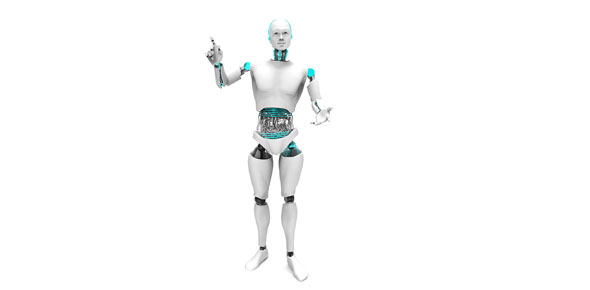# Robot C - Variables

11 Questions | Total Attempts: 187SettingsTo start the quiz, enter your LAST NAME FIRST and press start.

• 1.
The output of a sensor is always in the form of a…
• A.

Value.

• B.

Decimal.

• C.

Threshold.

• D.

Frequency.

• 2.
When a programmer “declares” a variable, they…
• A.

Shout out the value so the entire world can hear it.

• B.

Specify the type of value and give it a name.

• C.

Specify the aspect of the variable.

• D.

Create a line of code that will display a value.

• 3.
Which of the following is NOT a data type in Robot-C?
• A.

Integer

• B.

Floating Point Decimal

• C.

Value Assignment

• D.

String

• E.

Boolean

• 4.
Which of the following accurately describes a Boolean data type?
• A.

True or False statement

• B.

A string of typed characters (including letters, numbers, and/or typed symbols)

• C.

A numeric value that includes a decimal

• D.

Positive and negative whole numbers including zero

• 5.
Which of the following code declares the data type “Hello World!”?
• A.

Int

• B.

Float

• C.

String

• D.

Bool

• 6.
Which of the following variables are NOT part of an Automatic Threshold calculation program?
• A.

Int darkValue;

• B.

Int sumValue;

• C.

Int lightValue;

• D.

Int lineValue;

• E.

Int thresholdValue;

• 7.
What is the “lastSeen” variable used for in the Line Counting program?
• A.

To prevent the robot from counting the same line more than once

• B.

To give the robot a reference point to begin counting

• C.

To allow the robot to count the previous lines

• D.

To compile the number of lines crossed

• 8.
What keyword is used in Robot-C to begin the declaration of a function?
• A.

• B.

Func

• C.

Main

• D.

Void

• 9.
What behavior would running the following code cause your robot to exhibit? 1     void specialFunction(int p) 2     { 3       motor[motorC] = p/2; 4       motor[motorB] = p/2; 5       wait1Msec(100*p); 6     } 7 8     task main() 9     { 10   specialFunction(50); 11   }
• A.

The robot would move forward for 1 second at 50% motor power

• B.

The robot would move backwards for 1 second at 25% motor power

• C.

The robot would move forward for 5 seconds at 50% motor power

• D.

The robot would move forward for 5 seconds at 25% motor power

• 10.
What line(s) in the following code would you have to change to make the robot go forward for 5 seconds at 50% motor power? 1     void specialFunction(int p) 2     {           3       motor[motorC] = p/2; 4       motor[motorB] = p/2; 5       wait1Msec(100*p); 6     } 7 8     task main() 9     { 10   specialFunction(50); 11   }
• A.

Lines 3, 4, and 5

• B.

Line 1

• C.

Line 5 and 10

• D.

Line 3, 4, and 10

• 11.
Which of the following code declares the data type “43”?
• A.

Int

• B.

Float

• C.

String

• D.

Bool

Related TopicsBack to top